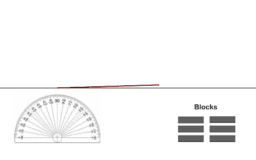# CCSS Gr. 4 Angles

Topic:
Geometry
Common Core Standards for Grade 4-Geometry Draw and identify lines and angles, and classify shapes by properties of their lines and angles. Draw points, lines, line segments, rays, angles (right, acute, obtuse), and perpendicular and parallel lines. Identify these in two-dimensional figures. Classify two-dimensional figures based on the presence or absence of parallel or perpendicular lines, or the presence or absence of angles of a specified size. Recognize right triangles as a category, and identify right triangles. Recognize a line of symmetry for a two-dimensional figure as a line across the figure such that the figure can be folded along the line into matching parts. Identify line-symmetric figures and draw lines of symmetry. Common Core Standards for Grade 4-Geometric measurement: understand concepts of angle and measure angles. Recognize angles as geometric shapes that are formed wherever two rays share a common endpoint, and understand concepts of angle measurement: --An angle is measured with reference to a circle with its center at the common endpoint of the rays, by considering the fraction of the circular arc between the points where the two rays intersect the circle. An angle that turns through 1/360 of a circle is called a “one-degree angle,” and can be used to measure angles. --An angle that turns through n one-degree angles is said to have an angle measure of n degrees. Measure angles in whole-number degrees using a protractor. Sketch angles of specified measure. Recognize angle measure as additive. When an angle is decomposed into non-overlapping parts, the angle measure of the whole is the sum of the angle measures of the parts. Solve addition and subtraction problems to find unknown angles on a diagram in real world and mathematical problems, e.g., by using an equation with a symbol for the unknown angle measure.
•### Define an Angle

•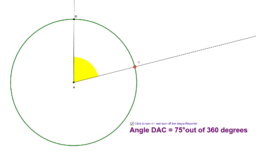### Measuring Angles on a Circle

•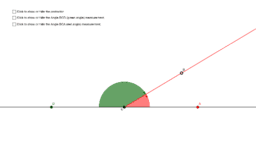### Introduction to the Protractor

•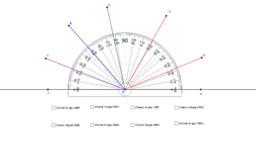### Practice with a Protractor

•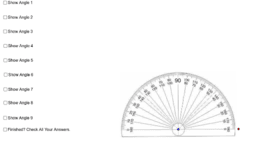### More Practice with the Protractor

•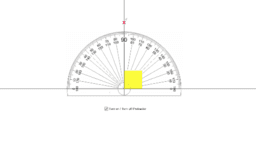### Estimating Angles

•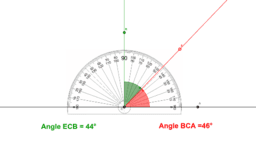### Complimentary Angles

•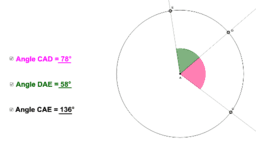•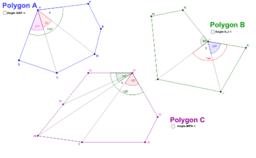### Find the Missing Angles

•